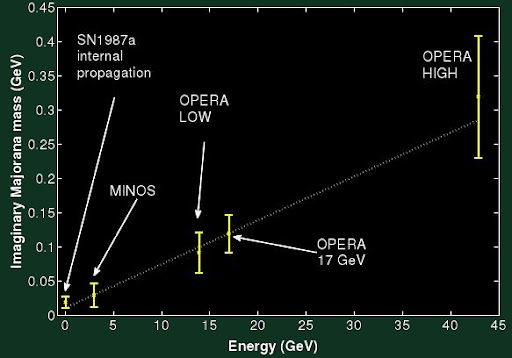Wednesday, October 12, 2011 ...//Majorana-Tamburini-Laveder superluminal neutrinosTommaso Dorigo invited Fabrizio Tamburini, a theorist behind questionable but eye-catching explanations, to write a guest blog:

Tamburini: Neutrinos Are Majorana Particles, Relativity Is OK (Science 2.0)

Apparent Lorentz violation with superluminal Majorana neutrinos at OPERA? (Tamburini-Laveder arXiv preprint)
The main "commercial" as well as "summary" of the work is the following intriguing graph:To agree with many of these bizarre experimental claims, the authors follow the mysteriously disappeared Ettore Majorana who added an equally mysterious imaginary mass term to the Dirac-Majorana equation for the neutrino in 1932.

First, let us forget the bizarre theory and discuss the phenomenology only. The graph suggests that you should add an imaginary energy-dependent term to the mass of the neutrino that goes like
$\delta m = i\cdot 0.007\cdot E/c^2$ Well, while this formula with an insanely huge numerical coefficient $$0.007$$ is compatible with several results, it's strange, indeed. First of all, we're used to the notion that the rest mass of the particles is energy-independent; on the contrary, the mass should control the energy-momentum relationship.

An ordinary energy-independent mass would produce a very different deviation of the speed $$v$$ from the speed of light in the vacuum $$c$$:
$E = \frac{m_0 c^2}{1-v^2/c^2} \,\Rightarrow \frac{v}{c}=\sqrt{1-\frac{m_0^2 c^4}{E^2}}$ If you Taylor-expand the last square root, you will see that the deviations of the speed $$v$$ from the speed of light should have corrections that drop with energy as $$1/E^2$$. Instead, to explain the superluminal neutrino experiments, assuming they're real, we need an energy-dependence that radically increases as a function of the energy.

Can this be real? What is the strange 1932 Majorana theory explaining these observations? You won't learn much from the 4-page preprint. But the claim is supposed to be that the large imaginary correction to the neutrino mass arises because of the interactions with a dense material.

The required correction seems to be huge and of course, I would instinctively dismiss this theory for many reasons that critics will probably agree about. However, one should also appreciated that neutrinos are very light particles (in the vacuum) so they naturally probe long distance scales.

Could it be that the neutrinos' propagation is this heavily affected by the rocks? I don't see a way how it could occur and I am convinced that relativity bans all such violations of the cosmic speed limit, whether or not you use rocks in your excuses why the ban should be lifted. Moreover, the neutrinos' interactions with the rocks just seem to be insanely weak according to everything we know so even neutrinos themselves should be mostly unaffected.

However, I will continue to think about it for a while because the graph is somewhat intriguing. Note that the neutrinos' mass scale is close to the fourth root of the vacuum energy density (the cosmological constant behind the "dark energy") so if we misunderstand something essential about this seemingly mundane energy scale, it could have far-reaching consequences, indeed.

Fixed speed

Incidentally, the obscure linear relationship above is simply equivalent to a fixed speed. Assume that the neutrinos in rocks always have the speed
$\frac{v}{c} = 1 + 2.5\times 10^{-5}$ where
$2.5\times 10^{-5} \approx \frac{1}{2} \times 0.007^2.$ Then
$E^2 - p^2 = E^2 - (1+2.5\times 10^{-5})^2 E^2 \approx -5\times 10^{-5} E^2$ and the square root of it is simply $$0.007\cdot i\cdot E$$, as previously indicated.

But why would the speed of neutrinos in rocks should be $$1.000025 c$$? Given seemingly super-weak interactions of the neutrinos with the matter, this required deviation from $$c$$ seems extremely strong. Moreover, the deviation has the wrong sign; causality should make all speeds in the materials slower than $$c$$, much like in the case of electromagnetism where the index of refraction doesn't drop below $$n=1$$.

Is there a loophole here? It seems incredibly unlikely to me but these matters should be carefully discussed. For example, someone should present the best proof she can of the general statement that the speed of neutrinos in a material is smaller than $$c$$. According to the conventional physics, the only effect of matter on neutrinos' propagation is the MSW effect which influences the oscillation patterns of neutrinos but not really the speed. To change the speed, you would need the two parts of the effective kinetic term for the Dirac-Majorana neutrino field to be rescaled by different factors whose ratio would have to be $$1.000025$$.

We think about neutrinos as being nearly non-interacting which could be a flawed illusion based on our low-energy perspective: near and above the electroweak scale, the neutrinos interact as much as the electrons do. However, the wrong (superluminal) sign of the speed correction is what seems truly impossible.

The GSI anomaly due to Rabi-like oscillations?

Another Italian preprint claims that the GSI anomaly, a non-exponentially decreasing number of decays of Pr-140 and Pm-142 as a function of time, may have an explanation in terms of Rabi0like oscillations. There are two modes of decay and the system oscillates in between them. Via arXiv blog.snail feedback (3) :

As Cherenkov was used in its affective determinations one has to ask too, what use Relativistic Muons as a determination of distant measures as to the nature of the density in those mass determinations?

The neutrino oscillation factor leaves room in that "distant measure" of [Proton Collision ->Decay to Muons and Muon Neutrinos ->Tau Neutrino ->...tau lepton may travel some tens of microns before decaying back into neutrino and charged tracks] to allocate the idea of an undelying structure depending on GRB measures as "Limiting properties of light and the universe with high energy photons from Fermi-detected Gamma Ray Bursts"

Those "four events" are tied to backdrop measures?

Best,Suerpluminals neutrinos

http://mathbin.net/74445